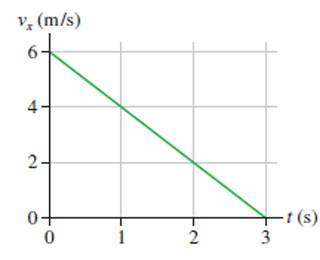Physics Practice Problems Velocity & Acceleration Graphs Practice Problems Solution: shows the velocity graph of a particle moving alo...

⚠️Our tutors found the solution shown to be helpful for the problem you're searching for. We don't have the exact solution yet.

# Solution: shows the velocity graph of a particle moving along the x-axis. Its initial position is x0 = 2.0 m at t0 = 0 s. You may want to review (Pages 44 - 48).At exttip{t}{t} = 1.0 s, what is the particles position?At exttip{t}{t} = 1.0 s, what is the particles velocity?At exttip{t}{t} = 1.0 s, what is the particles acceleration?

###### Problemshows the velocity graph of a particle moving along the x-axis. Its initial position is x0 = 2.0 m at t0 = 0 s.

You may want to review (Pages 44 - 48).

At = 1.0 s, what is the particles position?

At = 1.0 s, what is the particles velocity?

At = 1.0 s, what is the particles acceleration?

Velocity & Acceleration Graphs

Velocity & Acceleration Graphs

#### Q. The graph in shows the velocity of a motorcycle police officer plotted as a function of time.Find the instantaneous acceleration at t = 3 s.How far d...

Solved • Mon Oct 29 2018 18:49:57 GMT-0400 (EDT)

Velocity & Acceleration Graphs

#### Q. Three particles move along the x-axis, each starting at t0 = 0 s. The graph for A is a position-versus-time graph; the graph for B is a veloc...

Solved • Mon Oct 29 2018 17:46:58 GMT-0400 (EDT)

Velocity & Acceleration Graphs

#### Q. shows the position-versus-time graph for a moving object.At which lettered point or points is the object moving the fastest?At which lettered point o...

Solved • Mon Oct 29 2018 17:46:57 GMT-0400 (EDT)

Velocity & Acceleration Graphs

#### Q. FIGURE EX2.31 shows the acceleration-versus-time graph of a particle moving along the x-axis. Its initial velocity is v0x = 8.0 m/s at t 0 = 0 s. What...

Solved • Mon Oct 29 2018 17:46:32 GMT-0400 (EDT)